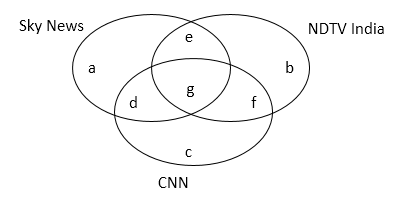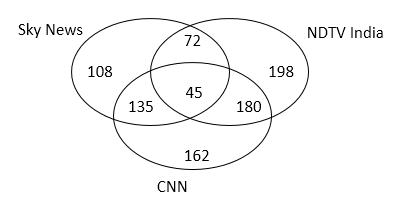# NIACL AO Mains– Quantitative Aptitude Questions Day- 01

Dear Readers, Bank Exam Race for the Year 2019 is already started, To enrich your preparation here we have providing new series of Practice Questions on Quantitative Aptitude – Section. Candidates those who are preparing for NIACL AO Mains 2019 Exams can practice these questions daily and make your preparation effective.

[WpProQuiz 5036]

Directions (1 – 5): Study the following information carefully and answer the questions given below:

There are total 900 students in a GK class. Each likes one or more among the three news channels viz. Sky News, NDTV India and CNN. 40% of the total number of students like Sky News. 18% of the total number of students like only CNN. 5% of the total number of students like all the three news channels. Respective ratio of number of students who like only CNN and total number of students who like CNN is 9:29. 15% of the total number of students like Sky News and CNN both but not NDTV India. 42% of the total number of students like NDTV India but not Sky News. 30% of the total number of students like NDTV India but not CNN.

1) Number of students who like Sky News and NDTV India both but not CNN is what percent of the number of students who like NDTV India and CNN both but not Sky News?

a) 30%

b) 40%

c) 35%

d) 25%

e) None of these

2) Out of the total number of students who like only Sky News, respective ratio of boys and girls is 5:4 and out of the total number of students who like only NDTV India, respective ratio of boys and girls is 6:5. Number of boys who like only Sky News is approximately what percent of the number of boys who like only NDTV India?

a) 52%

b) 48%

c) 62%

d) 56%

e) 39%

3) Find the respective ratio of total number of students who like Sky News but not CNN and total number of students who like CNN but not Sky News.

a) 11: 17

b) 13: 21

c) 15: 23

d) 10: 19

e) None of these

4) Find the difference between total number of students who like only one news channel and total number of students who like only two news channels.

a) 105

b) 61

c) 81

d) 97

e) None of these

5) Find the average of the total number of students who like NDTV India and CNN both but not Sky News and total number of students who like Sky News and NDTV India both but not CNN.

a) 144

b) 78

c) 126

d) 88

e) None of these

Directions (6 – 10): What value should come at the place of question mark in the following questions?

6) 11, 19, 65, 259, 1161, ?

a) 3081

b) 6021

c) 4421

d) 5041

e) None of these

7) 8, 50, 295,?, 5881, 17641

a) 1251

b) 1471

c) 1081

d) 1391

e) None of these

8) 10, 15, 35, 82.5,?, 562.5

a) 215

b) 210

c) 205

d) 220

e) None of these

9) 1000, 501, 170, 47.5,?

a) 14.5

b) 15

c) 16

d) 16.5

e) None of these

10) 11, 28, 39, 104,? , 276

a) 200

b) 75

c) 105

d) 64

e) None of these

Directions (1 – 5):a + b + c + d + e + f + g = 900 ———— (i)

a + d + e + g = 40/100 x 900 = 360 ————— (ii)

c = 18/100 x 900 = 162 ————- (iii)

g = 5/100 x 900 = 45 ———— (iv)

c + d + f + g = 29/9 x 162 = 522 ———– (v)

d = 15/100 x 900 = 135 ———— (vi)

From (iii), (iv), (v) and (vi)

162 + 135 + f + 45 = 522

=> f = 522 – 342

=> f = 180 ————- (vii)

b + f = 42/100 x 900 = 378 ———— (viii)

From (vii) and (viii)

b + 180 = 378

=> b = 378 – 180

=> b = 198 ———– (ix)

b + e = 30/100 x 900 = 270 ——— (x)

From (ix) and (x)

198 + e = 270

=> e = 270 – 198 = 72

From (ii)

a + 135 + 72 + 45 = 360

=> a = 360 – 252

=> a = 108Required percentage = 72/180 x 100 = 40%

Number of boys who like only Sky News = 5/9 x 108 = 60

Number of boys who like only NDTV India = 6/11 x 198 = 108

Required percentage = 60/108 x 100 = 55.56% = 56% approx.

Required ratio = (108 + 72): (162 + 180)

= 180: 342

= 10:19

Total number of students who like only one news channel

= 108 + 198 + 162

= 468

Total number of students who like only two news channels

= 135 + 72 + 180

= 387

Required difference = 468 – 387 = 81

Required average = (180 + 72)/2

= 252/2

= 126

Direction (6-10) :

11 x 1 + 23 = 19

19 x 2 + 33 = 65

65 x 3 + 43 = 259

259 x 4 + 53 = 1161

1161 x 5 + 63 = 6021

8 x 7 – 6 = 50

50 x 6 – 5 = 295

295 x 5 – 4 = 1471

1471 x 4 – 3 = 5881

5881 x 3 – 2 = 17641

10 x 0.5 + 10 = 15

15 x 1 + 20 = 35

35 x 1.5 + 30 = 82.5

82.5 x 2 + 40 = 205

205 x 2.5 + 50 = 562.5

1000 ÷ 2 + 1 = 501

501 ÷ 3 + 3 = 170

170 ÷ 4 + 5 = 47.5

47.5 ÷ 5 + 7 = 16.5

10 + 12 = 11

20 + 23 = 28

30 + 32 = 39

40 + 43 = 104

50 + 52 = 75

60 + 63 = 276